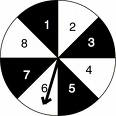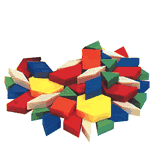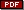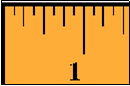Fractions

Fraction Models

Students need many concrete experiences with fractions to develop a deep understanding of the three models of fractions: area, linear and set models. Teachers need to address all three models in well-designed instructional activities so that students develop a rich concept of fractions that they can use to make sense of numbers, operations, measurement and probability.

Area Model: students divide shapes into the appropriate number of equal sections given by the denominator, then shade in the appropriate number of sections, given by the numerator to create a picture of the fraction. Equivalent fractions are also developed using this area model. Teachers may use any of these manipulatives to develop the area model:• pattern blocks
• circular fraction regions
• pizzas
• geoboards
• spinners
•Folded fraction pieces

Measurement or Linear Model: students use the number line model found on rulers or divide fraction strips into the appropriate sections by length. Teachers may use any of these manipulatives to develop the measurement or linear model:• number line
• rulers